Blender Python Script:  Mesh Manipulation,  (Part 5): Manufacturing Potatoids.

Version Française
 Main Index                                Python Index Previous Python (4): automating face creation Python: Menu and Opengl (6) Next
This slim and distinguished form is none other than a "Potatoid". I.e. a volume derived from the equation of a sphere. Its creation is not especially difficult, just utilize  the block concerning the creation of vertex co-ordinates in the previous examples.

 ... for i in range(0, n):        for j in range(0, n):              v=NMesh.Vert( i, j, 0 )              me.verts.append(v)  ...

We have just assigned the value of i to the co-ordinate X, and the value of j to Y.  A variable is added for the radius, and three other variables : x, y, and z ; in which we will store the result of the formulas. The following modifications make it possible to obtain a true sphere.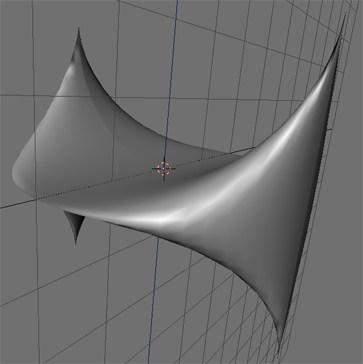... # radius of the sphere radius=2.0 for i in range(0, n):        for j in range(0, n):             x = sin(j*pi*2/(n-1))*cos(-pi/2+i*pi/(n-1))*radius             y = cos(j*pi*2/(n-1))*cos(-pi/2+i*pi/(n-1))*radius             z = sin(-pi/2+i*pi/(n-1))*radius        v=NMesh.Vert( x , y , z )        me.verts.append(v)  ...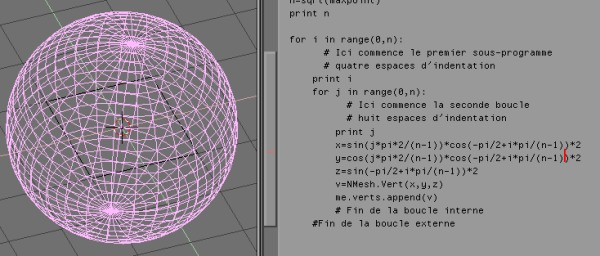The previously presented form is obtained by a very slight modification to the calculation of y

 ...    x= sin(j*pi*2/(n-1))*cos(-pi/2+i*pi/(n-1))*radius    y= cos(j*pi*2/(n-1)) * (1 -cos(-pi/2+i*pi/(n-1)) ) * radius    z= sin(-pi/2+i*pi/(n-1))*radius ...

The gate is now opened for more experiments and discoveries.

To obtain the file potatoid.py

 The great potatoids contest of the Little French's Tutorials During all the 2000's summer, for the greatest glory of Blender and the python, you are cordially invite to take part in the most incredible potatoid's contest . Let send your propositions, no picture!!, only the formula script, to:  jmsoler@free.fr Concurents formule Image Timmy "mangled shell" x=2*(1-2.718**((0.589*i)/(6*pi)))*cos(0.589*i)*(cos(0.5*0.196*j))**2 y= 2*(-1+2.718**((0.589*i)/(6*pi)))*cos(0.5*0.196*j)*cos(0.5*0.196*j)*sin(0.589*i) z=1-2.718**((0.589*i)/(3*pi))-sin(0.916*j)+2.718**((0.589*i)/(6*pi))*sin(0.196*j)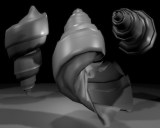Une espèce de  "Split Sea Shell"  x=2*(1-e**((0.5236*i)/(6*pi)))*cos(0.5236*i)*(cos(0.5*0.1745*j))**2 y=2*(-1+e**((0.5236*i)/(6*pi)))*cos(0.5*0.1745*j)*cos(0.5*0.1745*j)*sin(0.5236*i) z=1-e**((0.5236*i)/(3*pi))-sin(0.1745*j) + e**((0.5236*i)/(6*pi))*sin(0.1745*j) Make a display of the script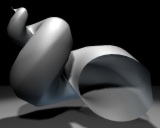thething s1=sin(j*pi*2/(n-1)) c2=cos(-pi/2+i*pi/(n-1)) c1=cos(j*pi*2/(n-1)) s2=sin(-pi/2+i*pi/(n-1)) "good pillow" x=s1*c2*2 y=c1*c2*2*0.5 z=s2*c1*2 Make a display of the script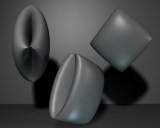"mulder's nightmare" x=s1*c2*2 y=c1*c2*2 z=s2*c1*s1*2 Make a display of the script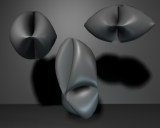"mulder's dream" x=s1*c2*2 y=c1*c2*2 z=s2*c1*s1*2 Make a display of the script"mulder's dream variation" x=s1*c2*2 y=c1*c2*2 z=s2*c1*s1*2 Make a display of the script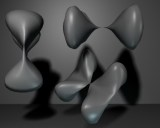"sexy saddle" x=s1*c2*2 y=c1*c2*2 z=s2*c1*s1*2 Make a display of the script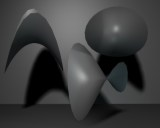"mechanical flower" x=s1*c2*2 y=c1*c2*2 z=s2*c1*s1*2 Make a display of the script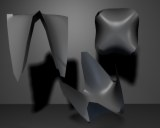"the lone star" x=s1*c2*2 y=c1*c2*2 z=s2*c1*s1*2 Make a display of the script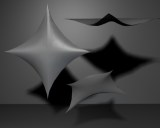. jö "noeud de trèfle" Not exactly a potatoid, but the contruction of the mesh is, quite, identical.  Afficher le scriptPrevious Python (4): automating face creation Python (6): Menu and Opengl Next Top of page

Les questions concernant cette page  peuvent être posées sur  :
news://news.zoo-logique.org/3D.Blender